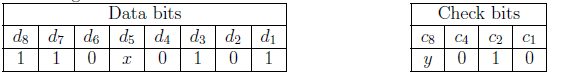# GATE | GATE CS 2021 | Set 1 | Question 39

• Last Updated : 09 Sep, 2021

Assume that a 12-bit Hamming codeword consisting of 8-bit data and 4 check bits is d8d7d6d5c8d4d4d3d2c4d1c2c1, where the data bits and the check bits are given in the following tables:Which one of the following choices gives the correct values of x and y?
(A) x is 0 and y is 0
(B) x is 0 and y is 1
(C) x is 1 and y is 0
(D) x is 1 and y is 1

Explanation:

A 12-bit Hamming codeword is given:

Check for C1

`c1 + d7 + d5 + d4 + d2 + d1 : 0 + 1 + x + 0 + 0 + 1 `

x=0 ( for even parity)

Check for C8

`c8 + d7 + d6 + d5 : y + 1 + 1 + 0 + 0`

y=0 ( for even parity)

So, option (A) is correct.

My Personal Notes arrow_drop_up
Recommended Articles
Page :